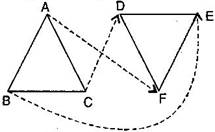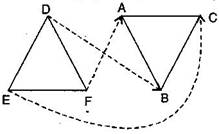### Congruence of Triangles - Solutions 1

CBSE Class –VII Mathematics
NCERT Solutions
Chapter 7 Congruence of Triangles
(Ex. 7.1)

Question 1. Complete the following statements:
1. Two line segments are congruent if _______________.
2. Among two congruent angles, one has a measure of ${70}^{\circ },$ the measure of other angle is _______________.
3. When we write $\mathrm{\angle }$A = $\mathrm{\angle }$B, we actually mean _______________.
Answer: (a) they have the same length
(b) ${70}^{\circ }$
(c) $m\mathrm{\angle }$A = $m\mathrm{\angle }$B
Question 2. Give any two real time examples for congruent shapes.
(ii) Two teacher’s tables
Question 3. If $\mathrm{\Delta }$ABC $\cong \mathrm{\Delta }$FED under the correspondence ABC $↔$ FED, write all the corresponding congruent parts of the triangles.
Answer: Given: $\mathrm{\Delta }$ABC $\cong$$\mathrm{\Delta }$FED.The corresponding congruent parts of the triangles are:
(i) $\mathrm{\angle }$$↔$$\mathrm{\angle }$F
(ii) $\mathrm{\angle }$$↔$$\mathrm{\angle }$E
(iii) $\mathrm{\angle }$$↔$$\mathrm{\angle }$D
(iv) $\overline{\text{AB}}$$↔$$\overline{\text{FE}}$
(v) $\overline{\text{BC}}$$↔$$\overline{\text{ED}}$
(vi) $\overline{\text{AC}}$$↔$$\overline{\text{FD}}$
Question 4. If $\mathrm{\Delta }$DEF $\cong \mathrm{\Delta }$BCA, write the part(s) of $\mathrm{\Delta }$BCA that correspond to:
i.$\mathrm{\angle }$E
ii.$\overline{\text{EF}}$
iii.$\mathrm{\angle }$F
iv.$\overline{\text{DF}}$
Answer: Given: $\mathrm{\Delta }$DEF $\cong$$\mathrm{\Delta }$BCA.(i) $\mathrm{\angle }$$↔$$\mathrm{\angle }$C
(ii) $\overline{\text{EF}}$$↔$$\overline{\text{CA}}$
(iii) $\mathrm{\angle }$$↔$$\mathrm{\angle }$A
(iv) $\overline{\text{DF}}$$↔$